### 3. REDSHIFT

The redshift z of an object is the fractional doppler shift of its emitted light resulting from radial motion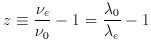(8)

where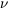0 and0 are the observed frequency and wavelength, ande ande are the emitted. Redshift is related to radial velocity v by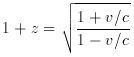(9)

where c is the speed of light. Many feel that it is wrong to view relativistic redshifts as velocities (eg, Harrison 1993), but I simply do not agree. The difference between an object's measured redshift and its cosmological redshift is due to its (radial) peculiar velocity; ie, we define the cosmological redshift as that part of the redshift due solely to the expansion of the Universe, or Hubble flow. For small v / c, or small distance d, in the expanding Universe, the velocity is linearly proportional to the distance (and all the distance measures, eg, angular diameter distance, luminosity distance, etc, converge)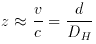(10)

where DH is the Hubble distance (see above). But this is only true for small redshifts!

(It is very important to note that galaxy redshift surveys, when presenting redshifts as radial velocities, always use the non-relativistic approximation v = c z, even when it is not appropriate physically; eg., Fairall 1992.)

In terms of cosmography, the cosmological redshift is directly related to the scale factor a (t), or the ``size'' of the Universe. For an object at redshift z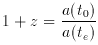(11)

where a (t0) is the size of the Universe at the time the light from the object is observed, and a (te) is the size at the time it was emitted.

Redshift is almost always determined with respect to us (or the frame centered on us but stationary with respect to the microwave background), but it is possible to define the redshift z12 between objects 1 and 2, both of which are cosmologically redshifted relative to us: the redshift z12 of an object at redshift z2 relative to a hypothetical observer at redshift z1 < z2 is given by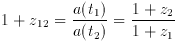(12)